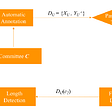# Pattern Based Chess Engine: Part 1

Standard Chess Engine usually involve the use of a board evaluation function. I approach to the problem of chess AI by creating a sequence evaluation function. The AI learns which sequence results in a win, and tries to replicate them in a game. This is unlike any other Chess Engine that exist out there (in my knowledge). So let’s see if it works.

In my previous posts I discuss the idea of treating chess as a language, where the moves can be considered as words in a language and a game sequence a sentence. In summary, I tested both generative and discriminative models to either generate the best move or classify whether a sub sequence of chess game will result in a win. While generative model didn’t perform well, the discriminative model was able to successfully find patterns that allowed a player to win a game.

In order to exploit this information, I build a simple chess engine that evaluates the possible sequences that occur during the course of a game and choose the best game sequence.

I use the CCRL 40/40 data set which consist of over and about 1 million games. The first step is the extract game sequences from the PGN files with their corresponding outcomes. The data set is of the form:

`[Event "CCRL 40/40"][Site "CCRL"][Date "2005.12.20"][Round "1.1.1"][White "Gandalf 6"][Black "Fritz 9"][Result "1-0"][ECO "D85"][Opening "Gruenfeld"][Variation "modern exchange variation"][PlyCount "83"][WhiteElo "2633"][BlackElo "2743"]1. d4 Nf6 2. c4 g6 3. Nc3 d5 4. cxd5 Nxd5 5. e4 Nxc3 6. bxc3 Bg7 7. Nf3 c5 8. Rb1 O-O 9. Be2 cxd4 10. cxd4 Qa5+ 11. Qd2 Qxd2+ 12. Bxd2 b6 13. O-O Bb7 14. Bd3 Rd8 15. Be3 Bxd4 16. Nxd4 e5 17. Nb5 Rxd3 18. Nc7 Nc6 19. Nxa8 Bxa8 20. Rfd1 Rc3 21. Rbc1 Rxc1 22. Bxc1 Kg7 23. f3 Kf6 24. Ba3 Nd4 25. Bb2 Nc6 26. Rd5 Ke6 27. Ba3 f5 28. Rd6+ Kf7 29. exf5 gxf5 30. h4 a5 31. h5 b5 32. Rd7+ Kf6 33. Rxh7 b4 34. Rh8 bxa3 35. Rg8 Kf7 36. Rxa8 Nd4 37. Kf2 f4 38. Rxa5 Nc6 39. Rxa3 Nb4 40. Ra7+ Kg8 41. a4 Nd3+ 42. Kg1 1-0`

I use the following code to extract the data from the PGN file. The trick is to read each line, ignore lines starting with ‘[‘ and ‘\n’, if it doesn’t, that’s the start of a game sequence. Store the game sequence until it encounter ‘\n’ again. That’s one game.Then I parse the game, drop ply counts.

`import redef get_general_data(stored_game):    outcomes = []    games = []    for i in range(len(stored_game)):        if stored_game[i][-1] == '0' or stored_game[i][-1] == '1': # or stored_game[i][-1] == '1':            game = re.sub(r'\d*\. ?', "", stored_game[i][:-3].rsplit(' ', 1))            outcome = stored_game[i][-3:].split('-')            outcomes.append(outcome.split('-'))            games.append(game)    file = open('dataset.txt', 'w+')    for i in range(len(games)):        file.write(games[i] + ' ' + outcomes[i] + '\n')    file.close()    return(len(games))if __name__ == '__main__':    file = open('CCRL.pgn', 'r')    game = ''    stored_game = []    for i in range(12500000):         line = file.readline()        if line != '\n' and line != '[':  # found game sequence            game = line            while True:                forseq = file.readline()                if forseq != '[':                    game += forseq                else:                    break            stored_game.append(game.replace('\n', ' ')[:-2])            game = ''print(get_general_data(stored_game))`

I only consider games where white either looses on wins the game.

Now I have the data in this form:

`d4 Nf6 c4 g6 Nc3 d5 cxd5 Nxd5 e4 Nxc3 bxc3 Bg7 Nf3 c5 Rb1 O-O Be2 cxd4 cxd4 Qa5+ Qd2 Qxd2+ Bxd2 b6 O-O Bb7 Bd3 Rd8 Be3 Bxd4 Nxd4 e5 Nb5 Rxd3 Nc7 Nc6 Nxa8 Bxa8 Rfd1 Rc3 Rbc1 Rxc1 Bxc1 Kg7 f3 Kf6 Ba3 Nd4 Bb2 Nc6 Rd5 Ke6 Ba3 f5 Rd6+ Kf7 exf5 gxf5 h4 a5 h5 b5 Rd7+ Kf6 Rxh7 b4 Rh8 bxa3 Rg8 Kf7 Rxa8 Nd4 Kf2 f4 Rxa5 Nc6 Rxa3 Nb4 Ra7+ Kg8 a4 Nd3+ Kg1 1`

The code is not as robust, as I have to manually enter the number of lines I want to scan to get some P number of games. But it gets the job done so it’s good enough.

But the data needs to be tokenized before passing it to the neural network. Also the above sequence doesn’t capture whether a move is played by white or black, example[c4 g6]. I order to overcome the problem, I simply prepend the white moves with ‘@’ and black moves with ‘~’ and the tokenizer lowers all the letters making the ‘@’ and ‘~’ the only basis of distinction. I fit the keras tokenizer and save it, so I can use in the game.

`file = open('dataset.txt', 'r')games = []outcomes = []for line in file.readlines():    x = line.strip('\n').split(' ')    games.append(x[:-1])    outcomes.append(int(x[-1]))#adding context to datasetfor i in range(len(games)):    for j in range(0, len(games[i])):        if j % 2 == 1:            games[i][j] = '~' + games[i][j]        else:            games[i][j] = '@' + games[i][j]import pandas as pdimport pickledata = pd.DataFrame({'game': games, 'outcome': outcomes})X, y = (data['game'].values, data['outcome'].values)from keras.preprocessing.text import Tokenizer# from keras.preprocessing.sequence import pad_sequencestk = Tokenizer(filters=' ', lower=True, oov_token=0)tk.fit_on_texts(X)f = open('tokenized.pkl', 'wb')pickle.dump(tk, f)X_seq = tk.texts_to_sequences(X)`

Next up: Generalizing the classification model.

In my previous post, I pick a sub sequence of fixed length T at either the end or start of the game and truncate the rest. The data set that it formed didn’t capture all the the possible scenarios that might occur during a game.

For example, at the starting of the game or in between, when the number of moves played is less than the length of time window, say len(T) = 5:

A Game sequence will be as following as the game progresses:

past_moves = [0, 0, 0, 0, 0] + next_move

past_moves = [0, 0, 0, 0, ‘e4’] + next_move

past_moves = [0, 0, 0, ‘e4’, ‘d7’] + next_move

and so on..

In order to capture all such variations in the training set, I use the following code.

`# Generating the datasetimport numpy as npimport randomno_games = 0maxlen = 50print(maxlen)X_padded = []Y_padded = []while no_games < 1000000:    this_game = random.randint(0, len(X_seq)-1)    game = X_seq[this_game]    # move = random.choice(range(maxlen, len(game) - 1))    # game_strip = game[move-maxlen: move]    move = random.choice(range(0, len(game) - 1))     #maxlen    if move - maxlen < 0:        game_strip =  * maxlen        for i in range(move + 1):            game_strip[maxlen - i - 1] = game[move - i]    else:        game_strip = game[move - maxlen:move]    # print(str(game_strip) + ' ' + str(y[this_game]))    X_padded.append(game_strip)    Y_padded.append(y[this_game])    no_games += 1`

What it essentially does is, it picks up a random game from the data set, picks a random move in the random game, and strip the previous n moves from the index selected n = len(Time_window). If the i-th move picked from the random game is less than the length of time window, take 0–ith move, and pad the rest.

After all the jazz, this is the data set looks like this. maxlen = 10

[0, 0, 0, 0, 0, 0, 0, 0, 5, 15] 1
[219, 400, 1363, 53, 32, 1384, 425, 1096, 586, 592] 0
[0, 4, 1, 8, 17, 3, 11, 109, 166, 5] 1
[5, 10, 2, 9, 98, 11, 176, 455, 4, 102] 0
[233, 133, 223, 178, 180, 243, 194, 248, 431, 126] 1
[10, 32, 1593, 964, 911, 605, 85, 36, 893, 347] 0
[72, 2, 2043, 383, 99, 145, 23, 136, 2237, 288] 1
[159, 109, 34, 18, 506, 133, 131, 90, 400, 20] 0
[743, 733, 865, 24, 284, 800, 487, 136, 495, 321] 0
[0, 0, 0, 0, 0, 5, 10, 2, 40, 4] 0

The next step is simple:

Split the data into training set and test set, fit the model onto the data and get it going. The choice of neural network was based on similarities between Chess and language.

Chess is sequential: a normal feedforward neural network will not preserve the order and see the time window as combination of different moves. LSTM helps preserve the temporal dependency. Further, LSTM does not suffer from vanishing/exploding gradient problem compared to RNN.

`from sklearn.model_selection import train_test_splitX_train, X_test, y_train, y_test = train_test_split(np.array(X_padded), np.array(Y_padded), test_size = 0.25, random_state = 1)batch_size = 64# Generating the Modelfrom keras.models import Sequentialfrom keras.layers import Embedding, LSTM, Dense, CuDNNLSTM, CuDNNGRUvocabulary_size = len(tk.word_counts.keys())+1max_words = maxlenembedding_size = 64model = Sequential()model.add(Embedding(vocabulary_size, embedding_size, input_length=max_words))model.add(CuDNNLSTM(64, return_sequences=True))model.add(CuDNNLSTM(64, return_sequences=True))model.add(CuDNNLSTM(64, return_sequences=True))model.add(CuDNNLSTM(64, return_sequences=True))model.add(CuDNNLSTM(64, return_sequences=True))model.add(CuDNNLSTM(64, return_sequences=False))# model.add(CuDNNLSTM(128, return_sequences=True))# model.add(CuDNNLSTM(128, return_sequences=False))model.add(Dense(1, activation='sigmoid'))model.compile(loss='binary_crossentropy', optimizer='adam', metrics=['accuracy'])model.summary()# Validation & Early Stoppingfrom keras.callbacks import ModelCheckpoint, EarlyStoppingfilepath = 'discriminate_weights_good1mil50_64_64_6x64.hdf5'early_stop_checkpoint = EarlyStopping(monitor='val_loss', mode='min', verbose=1, patience=2, min_delta=0)save_checkpoint = ModelCheckpoint(filepath, monitor='val_loss', verbose=1, save_best_only=True, save_weights_only=False, mode='min', period=1)callbacks_list = [early_stop_checkpoint, save_checkpoint]# Training the Modelfoo = model.fit(X_train, y_train, validation_split=0.05, batch_size=batch_size, epochs=1, callbacks=callbacks_list)print(foo)# Testing the Modelscores = model.evaluate(X_test, y_test, batch_size=128, verbose=0)print("Test accuracy", scores)`

When training on a data set with 5 million samples, I was able to achieve a training accuracy of 74.36% and test accuracy of 73.77%. After tuning the parameters, I achieved an validation accuracy of > 75.4 %(which I had to stop midway, because final year project, cannot run two simultaneous code on GPU).

All the code is available on my github:

https://github.com/anuveshkumar/PatternBasedChessEngine

Next Part: The Chess Engine

https://medium.com/@anuvesh.kumar/pattern-based-chess-engine-part-2-583290611fda

--

--

--

## More from Anuvesh Kumar

Interested in AI, ML, Music and Poetry. Love the nature, like to travel and explore.

Love podcasts or audiobooks? Learn on the go with our new app.

## Negation cue detection using Conditional Random Fields.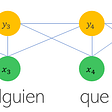## Review: MR-CNN & S-CNN — Multi-Region & Semantic-aware CNNs (Object Detection)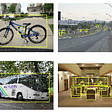## BASICS: Building a model which can identify diabetes## How I have built a self-driving car model## Top 10 model evaluation metrics for classification ML models## A Walkthrough of the Machine Learning Life Cycle## Calorie Estimation of Food Using ML And OpenCV## CenterNet: Objects as Points — A Comprehensive Guide## Anuvesh Kumar

Interested in AI, ML, Music and Poetry. Love the nature, like to travel and explore.

## Fast Classification and Clustering via Image Convolution Filters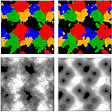## Processing of MQ-X sensors’ data with Machine Learning.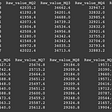## Optimization Algorithm : Gradient Descent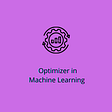## Acoustic Data Augmentation